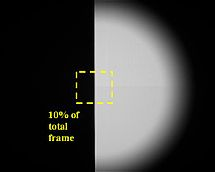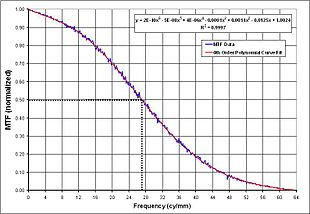# Modulation transfer function (infrared imaging)

Modulation transfer function (infrared imaging)

The Modulation Transfer Function (MTF) is used to approximate the position of best focus of an infrared imaging system. In an imaging system, best focus is typically achieved when the MTF is between 0.4 and 0.6; most often at 0.5 (50% cutoff frequency of the MTF) MTF is inversely related to the minimum resolvable temperature difference (MRTD), which is a measure of an infrared sensor's ability to resolve temperature difference. MTF is defined as the discrete fourier transform of the Line Spread Function (LSF). The LSF can be calculated by two different methods. One includes measuring the LSF directly from an idealized line approximation provided by an image of a slit target. The other involves differentiating the Edge Spread Function (ESF).

## ESF evaluationIn evaluating the ESF, an operator defines a box area equivalent to 10% of the total frame area of a knife-edge test target back-illuminated by a blackbody. The area is defined to encompass the edge of the target image.

An operator defines a box area encompassing the edge of a knife-edge test target image back-illuminated by a blackbody. The box area is defined to be approximately 10% of the total frame area. The image pixel data is translated into a two-dimensional array (pixel intensity and pixel position). The amplitude (pixel intensity) of each line within the array is normalized and averaged. This yields the edge spread function (ESF)$\text{ESF} = \frac{X - \mu}{\sigma} \qquad \qquad \sigma\, = \sqrt{\frac{\sum_{i=0}^{n-1} (x_i-\mu\,)^2}{n}} \qquad \qquad \mu\, = \frac{\sum_{i=0}^{n-1} x_i}{n}$

where

• ESF = the output array of normalized pixel intensity data
•$X\,$ = the input array of pixel intensity data
•$x_i\,$ = the ith element of$X\,$
•$\mu\,$ = the average value of the pixel intensity data
•$\sigma\,$ = the standard deviation of the pixel intensity data
•$n\,$ = number of pixels used in average

## LSF evaluation

The line spread function (LSF) can be found using two different methods. It can be found directly from an ideal line approximation provided by a slit test target or it can be derived from the ESF. Using the latter method, the LSF is defined as the first derivative of the ESF, which is differentiated using numerical methods.$\text{LSF} = \frac{d}{dx} \text{ESF}(x)$

Since the ESF can not be differentiated analytically, it is numerically approximated using the finite difference:$\text{LSF} = \frac{d}{dx}\text{ESF}(x) \approx \frac{\Delta \text{ESF}}{\Delta x}$$\text{LSF} \approx \frac{\text{ESF}_{i+1} - \text{ESF}_{i-1}}{2(x_{i+1} - x_i)}$

where

•$i\,$ = the index$i = 1,2,\dots,n-1$
•$x_i\,$ =$i^{th}\,$ position of the$i^{th}\,$ pixel
•$\text{ESF}_i\,$ = ESF of the$i^{th}\,$ pixel

## MTF Evaluation

The Modulation Transfer Function (MTF) is defined as the discrete fourier transform of the Line Spread Function. Thus, given the LSF, the MTF is approximated numerically. This data is graphed against the spatial frequency data. A sixth order polynomial is fitted to the MTF vs. spatial frequency curve to remove any trends. The 50% cutoff frequency is determined to yield the corresponding spatial frequency. Thus, the approximate position of best focus of the Unit Under Test is determined from this data.

### MTF calculations

The Fourier transform of the LSF can not be determined analytically by the following equations:$\text{MTF} = \mathcal{F} \left[ \text{LSF}\right] \qquad \qquad \text{MTF}= \int f(x) e^{-i 2 \pi\, x s}\, dx$

Therefore, the Fourier Transform is numerically approximated using the discrete Fourier transform$\mathcal{DFT}$.$\text{MTF} = \mathcal{DFT}[\text{LSF}] = Y_k = \sum_{n=0}^{N-1} y_n e^{-ik \frac{2 \pi}{N} n} \qquad k\in [0, N-1]$The MTF data versus spatial frequency is normalized by fitting a sixth order polynomial to it, making a smooth curve. The 50% cut-off frequency is determined and the corresponding spatial frequency is found, yielding the approximate position of best focus.

where

•$Y_k\,$ = the kth value of the MTF
•$N\,$ = number of data points
•$n\,$ = index
•$k\,$ = kth term of the LSF data
•$y_n\,$ =$n^{th}\,$ pixel position
•$i=\sqrt{-1}$

Since most computer software is not able to compute complex numbers directly, Euler's identity is implemented to break the transform into separate real and complex terms.$e^{\pm ia} = \cos(a) \, \pm \, i \sin(a)$$\text{MTF}= \mathcal{DFT}[\text{LSF}] = Y_k = \sum_{n=0}^{N-1} y_n \left[\cos\left(k\frac{2 \pi}{N} n\right) - i\sin\left(k \frac{2 \pi}{N} n\right)\right] \qquad k\in[0,N-1]$

The MTF is then plotted against spatial frequency and all relevant data concerning this test can be determined from that graph.

## See also

Wikimedia Foundation. 2010.

### Look at other dictionaries:

• Optical transfer function — The optical transfer function (OTF) of an imaging system (camera, video system, microscope etc) is the true measure of resolution (image sharpness) that the system is capable of. The common practice of defining resolution in terms of pixel count… …   Wikipedia

• Minimum resolvable temperature difference — (MRTD) is a measure for assessing the performance of infrared cameras, and is inversely proportional to the modulation transfer function. Typically, an operator is asked to assess the minimum temperature difference at which a 4 bar target can be… …   Wikipedia

• MTF — may refer to: * Make Trade Fair * Male to Female, a transwoman * Manual Transmission Fluid, see hydraulic fluid * Modulation Transfer Function, see optical transfer function * Modulation Transfer Function (Infrared Imaging) * Move to front… …   Wikipedia

• Optical resolution — This article is about optical resolution in optics. For the method of separating enantiomers in chemistry, see Chiral resolution. Optical resolution describes the ability of an imaging system to resolve detail in the object that is being imaged.… …   Wikipedia

• Mohammad Ataul Karim — Born May 4, 1953 (1953 05 04) (age 58) Sylhet, Bangladesh Citizenship Bangladesh / USA …   Wikipedia

• Depth of field — The area within the depth of field appears sharp, while the areas in front of and beyond the depth of field appear blurry …   Wikipedia

• Superlens — A superlens, super lens or perfect lens is a lens which uses metamaterials to go beyond the diffraction limit. The diffraction limit is an inherent limitation in conventional optical devices or lenses. In 2000, a type of lens was proposed,… …   Wikipedia

• spectroscopy — spectroscopist /spek tros keuh pist/, n. /spek tros keuh pee, spek treuh skoh pee/, n. the science that deals with the use of the spectroscope and with spectrum analysis. [1865 70; SPECTRO + SCOPY] * * * Branch of analysis devoted to identifying… …   Universalium

• electromagnetic radiation — Physics. radiation consisting of electromagnetic waves, including radio waves, infrared, visible light, ultraviolet, x rays, and gamma rays. [1950 55] * * * Energy propagated through free space or through a material medium in the form of… …   Universalium

• Active pixel sensor — An active pixel sensor (APS) is an image sensor consisting of an integrated circuit containing an array of pixel sensors, each pixel containing a photodetector and an active amplifier. There are many types of active pixel sensors including the… …   Wikipedia

### Share the article and excerpts

##### Direct link
Do a right-click on the link above
and select “Copy Link”